# Chapter 1 Number Systems RD Sharam Solution for Class 9th Maths

0
3027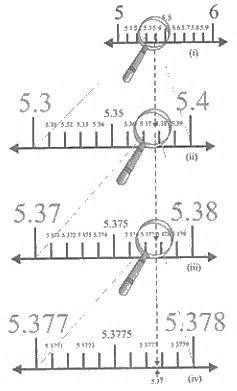# Chapter 1: Number System Exercise – 1.1

### Question: 1

Is 0 a rational number? Can you write it in the form P/Q, where P and Q are integers and Q ≠ 0?

### Solution:

Yes, 0 is a rational number and it can be written in P ÷ Q form provided that Q?

0 is an integer and it can be written various forms, for example

0 ÷ 2, 0 ÷ 100, 0 ÷ 95 etc.

### Question: 2

Find five rational numbers between 1 and 2

### Solution:

Given that to find out 5 rational numbers between 1 and 2

Rational number lying between 1 and 2= 3/2

= 1 < 3/2 < 2

Rational number lying between 1 and 3/2= 5/4

= 1 < 5/4 < 3/2

Rational number lying between 1 and 5/4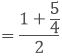Rational number lying between 3/2 and 2

= 9/8

= 1 < 9/8 < 5/4

Rational number lying between 3/2 and 2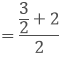= 7/4

= 3/2 < 7/4 < 2

Rational number lying between 7/4 and 2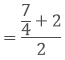= 15/8

= 7/4 < 15/8 < 2

Therefore, 1 < 9/8 < 5/4 < 3/2 < 7/4 < 15/8 < 2

### Question: 3

Find out 6 rational numbers between 3 and 4

### Solution:

Given that to find out 6 rational numbers between 3 and 4

We have,

3 × 7/7 = 21/7 and

4 × 6/6 = 28/7

We know 21 < 22 <  23 < 24 < 25 < 26 < 27 < 28

21/7 < 22/7 < 23/7 < 24/7 < 25/7 < 26/7 < 27/7 < 28/7

3 < 22/7 < 23/7 < 24/7 < 25/7 < 26/7 < 27/7 < 4

Therefore, 6 rational numbers between 3 and 4 are

22/7, 23/7, 24/7, 25/7, 26/7, 27/7

Similarly to find 5 rational numbers between 3 and 4, multiply 3 and 4 respectively with 6/6 and in order to find 8 rational numbers between 3 and 4 multiply 3 and 4 respectively with 8/8 and so on.

### Question: 4

Find 5 rational numbers between 3/5 and 4/5

### Solution:

Given to find out the 5 rational numbers between 3/5 and 4/5

To find 5 rational numbers between 3/5 and 4/5, 3/5 and 4/5 with 6/6

We have,

3/5 × 6/6 = 18/30

4/5 × 6/6 = 24/30

We know 18 < 19 < 20 < 21 < 22 <  23 < 24

18/30 < 19/30 < 20/30 < 21/30 < 22/30 < 23/30 < 24/30

3/5 < 1930 < 20/30 < 21/30 < 22/30 < 23/30 < 4/5

Therefore, 5 rational numbers between 3/5 and 4/5 are 19/30, 20/30, 21/30, 22/30, 23/30

### Question: 5

(i) Every whole number is a rational number

(ii) Every integer is a rational number

(iii) Every rational number is an integer

(iv) Every natural number is a whole number

(v) Every integer is a whole number

(vi) Every rational number is a whole number

### Solution:

(i)  True. As whole numbers include and they can be represented

For example – 0/10, 1/1, 2/1, 3/1….. And so on.

(ii) True. As we know 1, 2, 3, 4 and so on, are integers and they can be represented in the form of 1/1, 2/1, 3/1, 4/1.

(iii) False. Numbers such as 3/2, 1/2, 3/5, 4/5 are rational numbers but they are not integers.

(iv) True. Whole numbers include all of the natural numbers.

(v) False. As we know whole numbers are a part of integers.

(vi) False. Integers include -1, -2, -3 and so on….which is not whole number

# Chapter 1: Number System Exercise – 1.2

### Question: 1

Express the following rational numbers as decimals:

(i) 42/100

(ii) 327/500

(iii) 15/4

### Solution:

(i) By long division method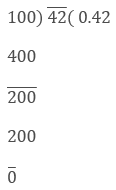Therefore, 42/100 = 0.42

(ii) By long division method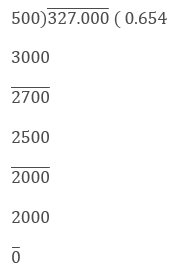Therefore, 327/500 = 0.654

(iii) By long division method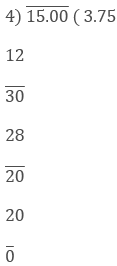Therefore, 15/4 = 3.75

### Question: 2

Express the following rational numbers as decimals:

(i)  2/3

(ii) – (4/9)

(iii) – (2/15)

(iv) – (22/13)

(v)   437/999

### Solution:

(i) By long division methodTherefore, 2/3 = 0.66

(ii) By long division method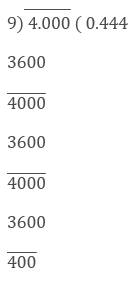Therefore, – 4/9 = – 0.444

(iii) By long division method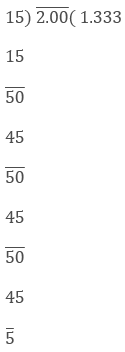Therefore, 2/15 = -1.333

(iv) By long division method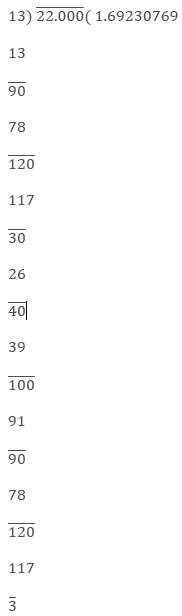Therefore, – 22/13 = – 1.69230769

(v) By long division methodTherefore, 437/999 = 0.43743

### Question: 3

Look at several examples of rational numbers in the form of p/q (q ≠ 0), where p and q are integers with no common factor other than 1 and having terminating decimal representations. Can you guess what property q must satisfy?

### Solution:

A rational number p/q is a terminating decimal

only, when prime factors of q are q and 5 only. Therefore,

p/q is a terminating decimal only, when prime

factorization of q must have only powers of 2 or 5 or both.

# Chapter 1: Number System Exercise – 1.3

### Question: 1

Express each of the following decimals in the form of rational number.

(i)  0.39

(ii) 0.750

(iii) 2.15

(iv) 7.010

(v)  9.90

(vi) 1.0001

### Solution:

(i) Given,

0.39 = 39/100

(ii) Given,

0.750 = 750/1000

(iii) Given,

2.15 = 215/100

(iv) Given, 9.101

(iv) Given,

7.010 = 7010/1000

(v) Given,

9.90 = 990/100

(vi) Given,

1.0001 = 10001/10000

### Question: 2

Express each of the following decimals in the form of rational number (p/q)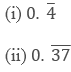### Solution: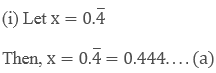Multiplying both sides of equation (a) by 10, we get,

10x = 4.44…. (b)

Subtracting equation (1) by (2)

9x = 4

x = 4/9

Hence,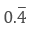= x = 4/9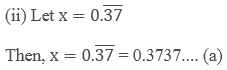Multiplying both sides of equation (a) by 100, we get,

100 x = 37.37….  (b)

Subtracting equation (1) by (2)

99 x = 37

x = 37/99

Hence,= x = 37/99

# Chapter 1: Number System Exercise – 1.4

### Question: 1

Define an irrational number.

### Solution:

An irrational number is a real number which can be written as a decimal but not as a fraction i.e. it cannot be expressed as a ratio of integers. It cannot be expressed as terminating or repeating decimal.

### Question: 2

Explain how an irrational number is differing from rational numbers?

### Solution:

An irrational number is a real number which can be written as a decimal but not as a fraction i.e. it cannot be expressed as a ratio of integers. It cannot be expressed as terminating or repeating decimal.

For example, 0.10110100 is an irrational number

A rational number is a real number which can be written as a fraction and as a decimal i.e. it can be expressed as a ratio of integers. . It can be expressed as terminating or repeating decimal.

For examples,

0.10 andboth are rational numbers

### Question: 3

Find, whether the following numbers are rational and irrational

(i) √7

(ii)  √4

(iii) 2 + √3

(iv) √3 + √2

(v) √3 + √5

(vi) (√2 – 2)2

(vii)  (2 – √2) (2 + √2)

(viii) (√2 + √3)2

(ix) √5 – 2

(x) √23

(xi) √225

(xii) 0.3796

(xiii) 7.478478…

(xiv) 1.101001000100001…..

### Solution:

(i) √7 is not a perfect square root so it is an Irrational number.

(ii) √4 is a perfect square root so it is an rational number.

We have,

√4 can be expressed in the form of

a/b, so it is a rational number. The decimal representation of √9 is 3.0. 3 is a rational number.

(iii) 2 + √3

Here, 2 is a rational number and √3 is an irrational number

So, the sum of a rational and an irrational number is an irrational number.

(iv) √3 + √2

√3 is not a perfect square and it is an irrational number and √2 is not a perfect square and is an irrational number. The sum of an irrational number and an irrational number is an irrational number, so √3 + √2 is an irrational number.

(v) √3 + √5

√3 is not a perfect square and it is an irrational number and √5 is not a perfect square and is an irrational number. The sum of an irrational number and an irrational number is an irrational number, so √3 + √5 is an irrational number.

(vi) (√2 – 2)2

We have, (√2 – 2)2

= 2 + 4 – 4√2

= 6 + 4√2

6 is a rational number but 4√2 is an irrational number.

The sum of a rational number and an irrational number is an irrational number, so (√2 + √4)2 is an irrational number.

(vii) (2 -√2) (2 + √2)

We have,

(2 – √2) (2 + √2) = (2)2 – (√2)2 [Since, (a + b)(a – b) = a2 – b2]

4 – 2 = 2/1

Since, 2 is a rational number.

(2 – √2)(2 + √2) is a rational number.

(viii) (√2 +√3)2

We have,

(√2 + √3)2 = 2 + 2√6 + 3 = 5+√6   [Since, (a + b)2 = a2 + 2ab + b2

The sum of a rational number and an irrational number is an irrational number, so (√2 + √3)2 is an irrational number.

(ix) √5 – 2

The difference of an irrational number and a rational number is an irrational number. (√5 – 2) is an irrational number.

(x) √23

√23 = 4.795831352331….

As decimal expansion of this number is non-terminating, non-recurring so it is an irrational number.

(xi) √225

√225 = 15 = 15/1

√225 is rational number as it can be represented in p/q form.

(xii) 0.3796

0.3796, as decimal expansion of this number is terminating, so it is a rational number.

(xiii) 7.478478……

7.478478 = 7.478, as decimal expansion of this number is non-terminating recurring so it is a rational number.

(xiv) 1.101001000100001……

1.101001000100001……, as decimal expansion of this number is non-terminating, non-recurring so it is an irrational number

### Question: 4

Identify the following as irrational numbers. Give the decimal representation of rational numbers:

(i) √4

(ii) 3 × √18

(iii) √1.44

(iv) √(9/27)

(v) – √64

(vi) √100

### Solution:

(i) We have,

√4 can be written in the form of

p/q. So, it is a rational number. Its decimal representation is 2.0

(ii). We have,

3 × √18

= 3 × √2 × 3 × 3

= 9×√2

Since, the product of a ratios and an irrational is an irrational number. 9 ×√2 is an irrational.

3 ×√18 is an irrational number.

(iii) We have,

√1.44

= √(144/100)

= 12/10

= 1.2

Every terminating decimal is a rational number, so 1.2 is a rational number.

Its decimal representation is 1.2.

(iv) √(9/27)

We have,

√(9/27)

=3/√27

= 1/√3

Quotient of a rational and an irrational number is irrational numbers so

1/√3 is an irrational number.

√(9/27) is an irrational number.

(v) We have,

-√64

= – 8

= – (8/1)

= – (8/1) can be expressed in the form of a/b,

so – √64 is a rational number.

Its decimal representation is – 8.0.

(vi) We have,

√100

= 10  can be expressed in the form of a/b,

So √100 is a rational number

Its decimal representation is 10.0.

### Question: 5

In the following equations, find which variables x, y and z etc. represent rational or irrational numbers:

(i) x2 = 5

(ii) y2 = 9

(iii) z2 = 0.04

(iv) u2 = 174

(v) v2 = 3

(vi) w2 = 27

(vii) t2 = 0.4

### Solution:

(i) We have,

x2 = 5

Taking square root on both the sides, we get

X = √5

√5 is not a perfect square root, so it is an irrational number.

(ii) We have,

= y2 = 9

= 3

= 3/1 can be expressed in the form of a/b, so it a rational number.

(iii) We have,

z2 = 0.04

Taking square root on the both sides, we get

z = 0.2

2/10 can be expressed in the form of a/b, so it is a rational number.

(iv) We have,

u2 = 17/4

Taking square root on both sides, we get,

u = √(17/4)

u = √17/2

Quotient of an irrational and a rational number is irrational, so u is an Irrational number.

(v) We have,

v2 = 3

Taking square root on both sides, we get,

v = √3

√3 is not a perfect square root, so v is irrational number.

(vi) We have,

w2 = 27

Taking square root on both the sides, we get,

w = 3√3

Product of a irrational and an irrational is an irrational number. So w is an irrational number.

(vii) We have,

t2 = 0.4

Taking square root on both sides, we get,

t = √(4/10)

t = 2/√10

Since, quotient of a rational and an Irrational number is irrational number. t2 = 0.4 is an irrational number.

### Question: 6

Give an example of each, of two irrational numbers whose:

(i) Difference in a rational number.

(ii) Difference in an irrational number.

(iii) Sum in a rational number.

(iv) Sum is an irrational number.

(v) Product in a rational number.

(vi) Product in an irrational number.

(vii) Quotient in a rational number.

(viii) Quotient in an irrational number.

### Solution:

(i) √2 is an irrational number.

Now, √2 -√2 = 0.

0 is the rational number.

(ii) Let two irrational numbers are 3√2 and √2.

3√2 – √2 = 2√2

5√6 is the rational number.

(iii) √11 is an irrational number.

Now, √11 + (-√11) = 0.

0 is the rational number.

(iv) Let two irrational numbers are 4√6 and √6

4√6 + √6

5√6 is the rational number.

(iv) Let two Irrational numbers are 7√5 and √5

Now, 7√5 × √5

= 7 × 5

= 35 is the rational number.

(v) Let two irrational numbers are √8 and √8.

Now, √8 × √8

8 is the rational number.

(vi) Let two irrational numbers are 4√6 and √6

Now, (4√6)/√6

= 4 is the rational number

(vii) Let two irrational numbers are 3√7 and √7

Now, 3 is the rational number.

(viii) Let two irrational numbers are √8 and √2

Now √2 is an rational number.

### Question: 7

Give two rational numbers lying between 0.232332333233332 and 0.212112111211112.

### Solution:

Let a = 0.212112111211112

And, b = 0.232332333233332…

Clearly, a < b because in the second decimal place a has digit 1 and b has digit 3 If we consider rational numbers in which the second decimal place has the digit 2, then they will lie between a and b.

Let. x = 0.22

y = 0.22112211… Then a < x < y < b

Hence, x, and y are required rational numbers.

### Question: 8

Give two rational numbers lying between 0.515115111511115 and 0. 5353353335

### Solution:

Let, a = 0.515115111511115…

And, b = 0.5353353335..

We observe that in the second decimal place a has digit 1 and b has digit 3, therefore, a < b.

So If we consider rational numbers

x = 0.52

y = 0.52062062…

We find that,

a < x < y < b

Hence x and y are required rational numbers.

### Question: 9

Find one irrational number between 0.2101 and 0.2222 … =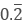### Solution:

Let, a = 0.2101 and,

b = 0.2222…

We observe that in the second decimal place a has digit 1 and b has digit 2, therefore a < b in the third decimal place a has digit 0.

So, if we consider irrational numbers

x = 0.211011001100011….

We find that a < x < b

Hence x is required irrational number.

### Question: 10

Find a rational number and also an irrational number lying between the numbers 0.3030030003… and 0.3010010001…

### Solution:

Let,

a = 0.3010010001 and,

b = 0.3030030003…

We observe that in the third decimal place a has digit 1 and b has digit

3, therefore a < b in the third decimal place a has digit 1. So, if we

consider rational and irrational numbers

x = 0.302

y = 0.302002000200002…..

We find that a < x < b and, a < y < b.

Hence, x and y are required rational and irrational numbers respectively.

### Question: 11

Find two irrational numbers between 0.5 and 0.55.

### Solution:

Let a = 0.5 = 0.50 and b = 0.55

We observe that in the second decimal place a has digit 0 and b has digit

5, therefore a < 0 so, if we consider irrational numbers

x = 0.51051005100051…

y = 0.530535305353530…

We find that a < x < y < b

Hence x and y are required irrational numbers.

### Question: 12

Find two irrational numbers lying between 0.1 and 0.12.

### Solution:

Let a = 0.1 = 0.10

And b = 0.12

We observe that In the second decimal place a has digit 0 and b has digit 2.

Therefore, a < b.

So, if we consider irrational numbers

x = 0.1101101100011… y = 0.111011110111110… We find that a < x < y < 0

Hence, x and y are required irrational numbers.

### Question: 13

Prove that √3 + √5 is an irrational number.

### Solution:

If possible, let √3 + √5 be a rational number equal to x.

Then,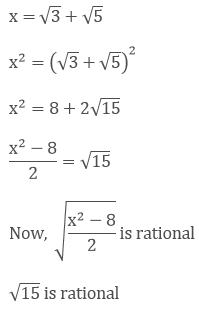Thus, we arrive at a contradiction.

Hence, √3 + √5 is an irrational number.

Previous articleClass 9th Math R.D. Sharma Solutions